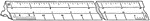Architect's Scale Ruler

An Architect's Scale ruler for drawing machinery and structures.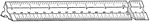Engineer's Scale Ruler

An Engineer's Scale ruler for plotting and map drawing.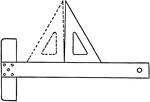Triangle Accuracy Test using T-square

A test to ensure the triangle is accurate by drawing a perpendicular line from a T-square.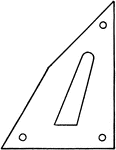Rondinella Triangle

The Rondinella triangle is a modified triangle ruler, where the hypotenuse is outwardly bent.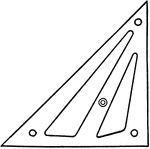Zange Triangle

The Zange triangle is a modified triangle ruler, where one of the legs is longer making the hypotenuse…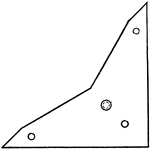Kelsey Triangles

The Kelsey triangle is a modified triangle ruler, where the hypotenuse is inwardly bent.